2.2 Franck-Condon Theory

Charge trapping models found in literature usually assume elastic electron tunneling, which ignores the atomic configuration of the defects. In the Franck-Condon theory , however, changes in the defect configuration play a crucial role in the charge trapping process. For instance, this theory predicts that the defect levels can be subject to a shift  (discussed in Section 2.2). In order to support the physical understanding of such trapping processes, one has to deal with the basics of microscopic theories, which provide the most complete description of a physical process, such as charge trapping.

Therefore, the Schrödinger equation  for a system involving electrons and nuclei is taken as a starting point. Its Hamiltonian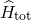can be formulated as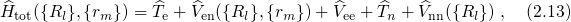where the subscripts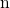and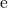refer to nuclei and electrons, respectively.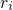and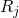are the coordinates of a certain electron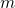or a certain nucleus, respectively, whereas the curly braces stand for the whole set of coordinates, such as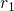,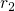,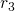,for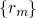. The Hamiltonian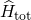contains all contributions from the electrons (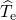) and nuclei (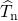) kinetic energies as well as from the Coulombic electron-electron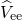, nucleus-nucleus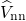, and electron-nucleus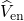interactions. Then, the Schrödinger equation reads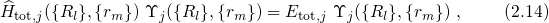where the energy of the whole system of the electrons and the nuclei is denoted as. It is important to note at this point that the wavefunctions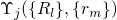depend on all positions of the electrons as well as the nuclei and thus the equation (2.14) cannot be solved due to its mathematical complexity.

In order to obtain an approximate solution, one usually employs the Born-Oppenheimer approximation, also known as the adiabatic approximation , which states that the electrons move much faster than the nuclei. In this picture, the electrons instantaneously adapt to each atomic configuration and the motion of the nuclei can be neglected for the solution of the electron system. Based on this assumption the wavefunction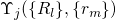can be split into an electron (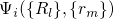) and a nuclei (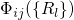) part.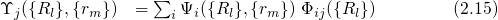In a further consequence, this ansatz separates the electron and nuclei system, which are then characterized by the so-called ‘electronic’ and ‘vibrational’ states, respectively. The electron system is described by the electronic Schrödinger equation with a fixed nuclei configuration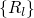.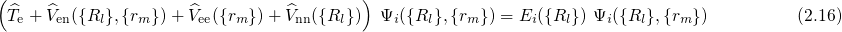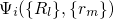stands for the many-electron wavefunction, which is a function of the electron coordinates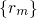. The nuclei have fixed positions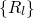and enter the equation (2.16) as parameters only. Consequently, their positive charges form an external potential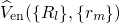to the electron system. The eigenvalues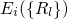of equation (2.16) re-enter the Schrödinger equation of the nuclei system,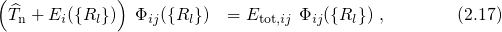and act as an external potential, in which the nuclei move. This potential is therefore referred to as the adiabatic potential or the potential energy surface. The nuclei in the Schrödinger equation (2.17) are treated as quantum mechanical particles. Therefore, they cannot be visualized as being to be located at certain points in space but are described by their wavefunctions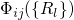. Furthermore, the energy spectrum of the nuclei system is quantized and includes discretized excitations known as lattice vibrations in crystals. In quantum chemistry, the nuclei system is frequently visualized in so-called configuration coordinate diagrams (see Fig. 2.2). The ordinate of these diagrams represents the total energy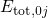presuming that the electron system is in its ground state. The abscissa is the configuration coordinate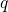, which summarizes the nuclei configurationin one quantity. It is important to note here that the coupling of the two Schrödinger equations (2.16) and (2.17) results in so-called ‘vibronic’ states, which are combinations of the electronic and the vibrational states and are defined by the wavefunctions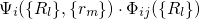and their corresponding energy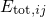.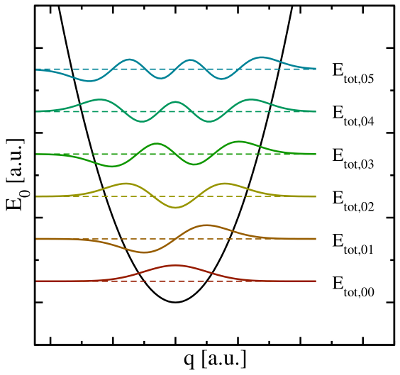Figure 2.2: The configuration coordinate diagram of a nuclei system in a parabolic adiabatic potential (black solid line). The ordinate represents the total energy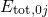, where the electron system is assumed to be in its ground state. The abscissa is the configuration coordinate, which describes the atomic configuration. The quantum mechanical nature of the nuclei is reflected in the formation of wavefunctions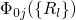(colored lines), each of which has its corresponding discrete energies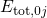.

According to the Franck-Condon principle , these vibronic transitions involve a change in the electronic as well as in the vibrational state. The corresponding rate can be calculated using Fermi’s golden rule (see Appendix A.1)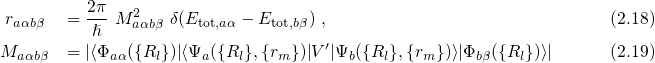with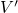being the perturbation operator.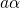and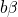denote the initial and the final vibronic state, respectively, where the latin and greek symbols refer to the electronic and vibrational states. According to the Franck-Condon approximation, the matrix elementcan be separated into two factors: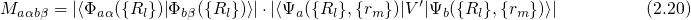Using the above equation, the transition rate (2.19) can be rewritten as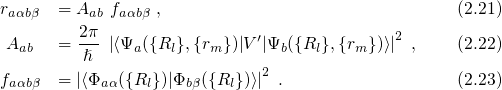Here,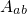denotes the electronic matrix element and is associated with an electronic transition, for instance electron or hole tunneling in the case of charge trapping in defects. The Franck-Condon factor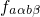gives the probability of the vibrational transition, which is determined by the overlap of the nuclei wavefunctions. The probability for such a transition is lower than that for the electron transition. Therefore,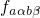becomes the decisive factor in equation (2.21).